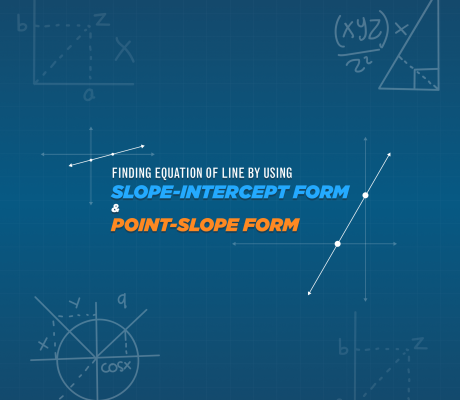# Educational Math Blogs### Finding The Equation Of The Line By Using The Slope-Intercept Form And Point-Slope Form

In mathematics and physics, equations of the line are used widely. Equation of the line is very essential in different branches of science and everyday problems. It is that equation in which the degree of each variable is one. This article will discuss the Slope-Intercept And Point-Slope of Linear Equations The equation of the line […]### Math Collaborative Learning

Some may consider online math education to be an individual venture. Imagine a student alone at their desk and trying to find all the answers on their own. With this kind of setup, it won’t help the students solve any problem. It may be the case for some teachers. Well, there are online institutions that […]